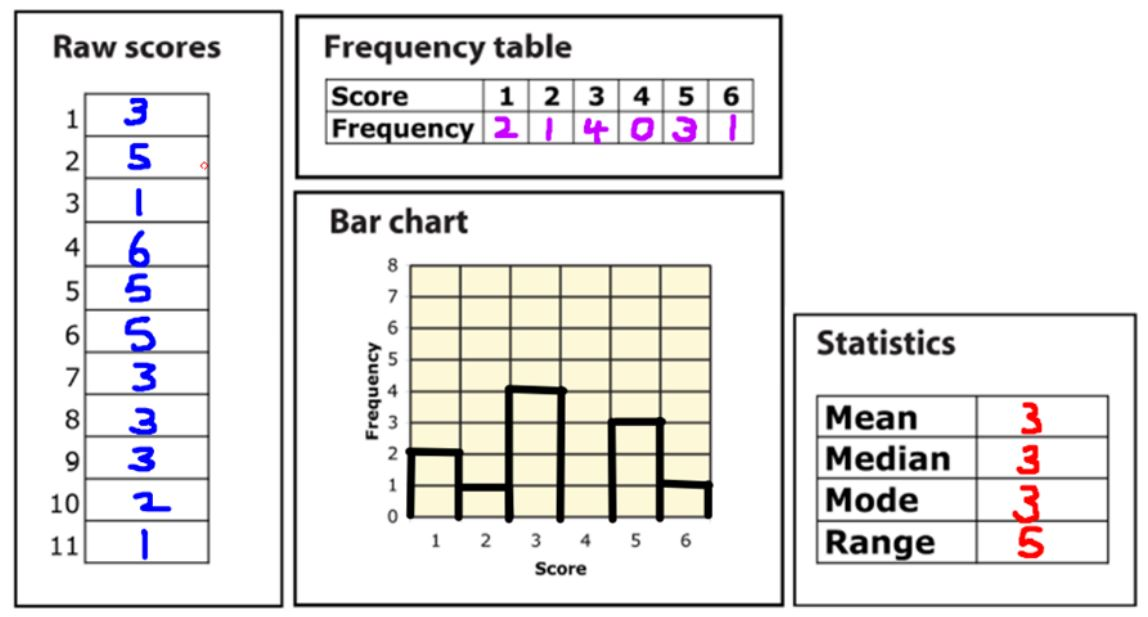S4 Understanding Mean, Median, Mode and Range

Main page – All Standards Unit ResourcesThe pdf for this activity can be found here.

The task is to match the bar charts with the statistics (mean, median, mode and range are all given). This has worked really well with my students and I feel leads to a deeper understanding than just simply calculating these statistics.

PowerPoint: S4 Understanding Mean Median Mode and Range

The PowerPoint here introduces the activity with an example to make sure students are clear on the problem and the calculation of the statistics. The first few slides show some dice throws which the students note down and create a frequency table from. The statistics are then calculated. The last part of the activity provides an extra challenge.

The solutions are all included in the PowerPoint.